# Sums and Averages

## How to Calculate Sums and Averages in Google Sheets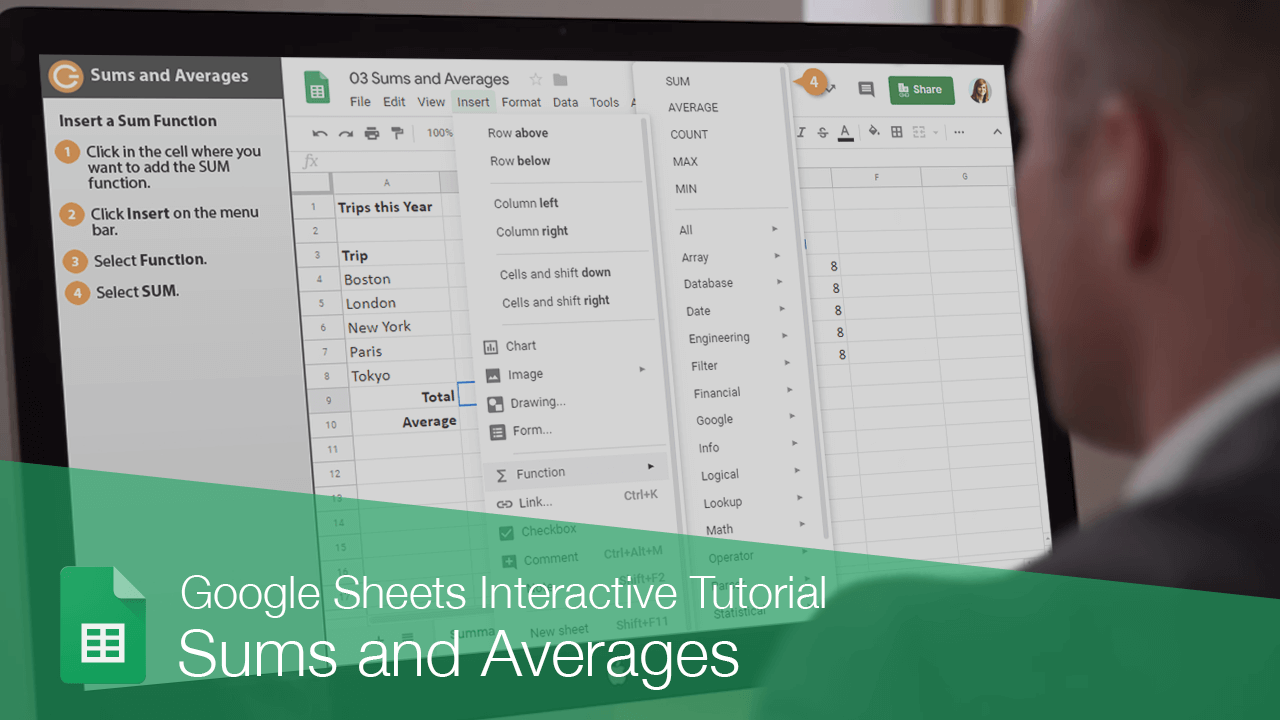You can use the SUM function to calculate totals in your spreadsheets.

There are two ways to insert a function. If you know the proper syntax, you can just type it in the cell as part of a formula. You can also select a function from a menu to insert it.

### Insert a SUM Function

Creating a sum formula is simple.

1. Click in the cell where you want to add the SUM function.
2. Click Insert on the menu bar.
3. Select Function.

This menu shows a few common functions, as well as submenus for plenty of function categories.

4. Select SUM.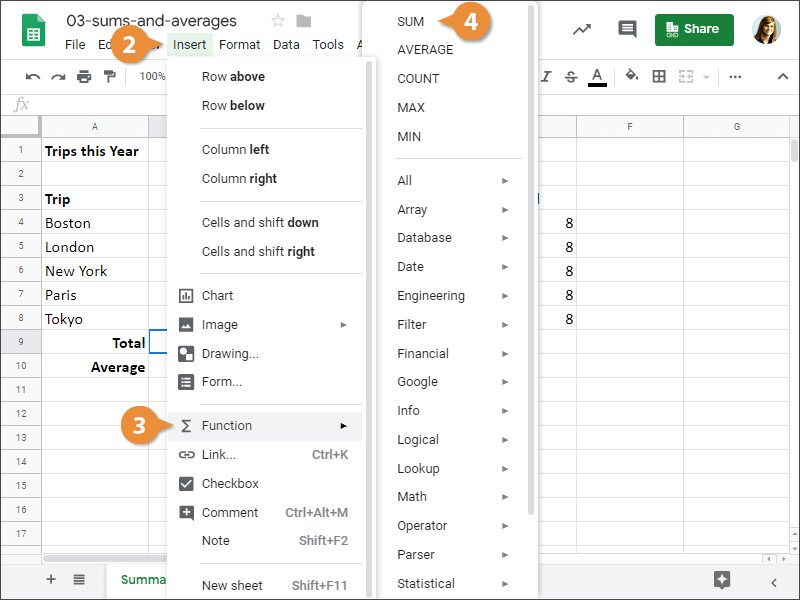A new formula is started in the selected cell, using the SUM function. Now, we just need to enter the cell range we want to use by typing it in, or selecting the range on the sheet.

5. Select the cells you want to find the sum for.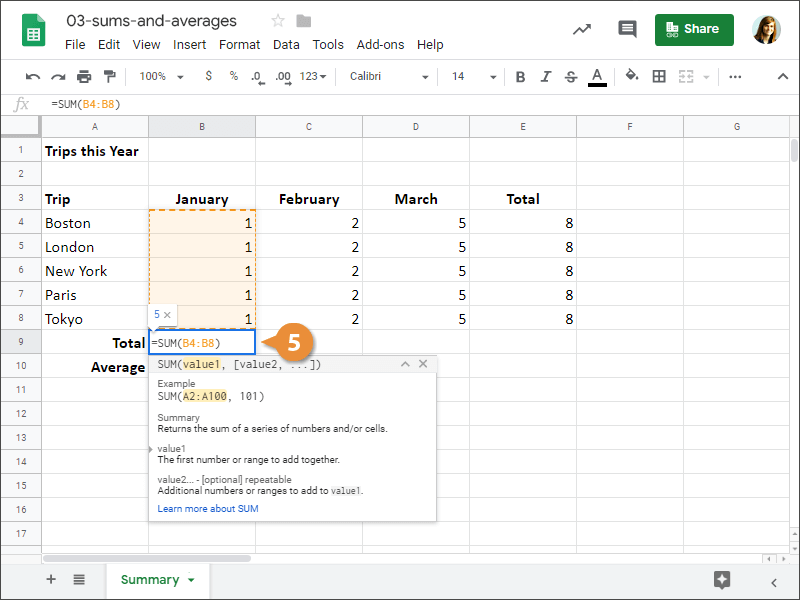6. Press Enter.

The sum of the selected cells is calculated and appears in the cell.

### Insert an AVERAGE Function

Next, let's calculate the average of a cell range. Calculating an average works similar to calculating a sum, just with a different function.

1. Click in the cell where you want to add the AVERAGE function.
2. Click Insert on the menu bar.
3. Select Function.
4. Select AVERAGE.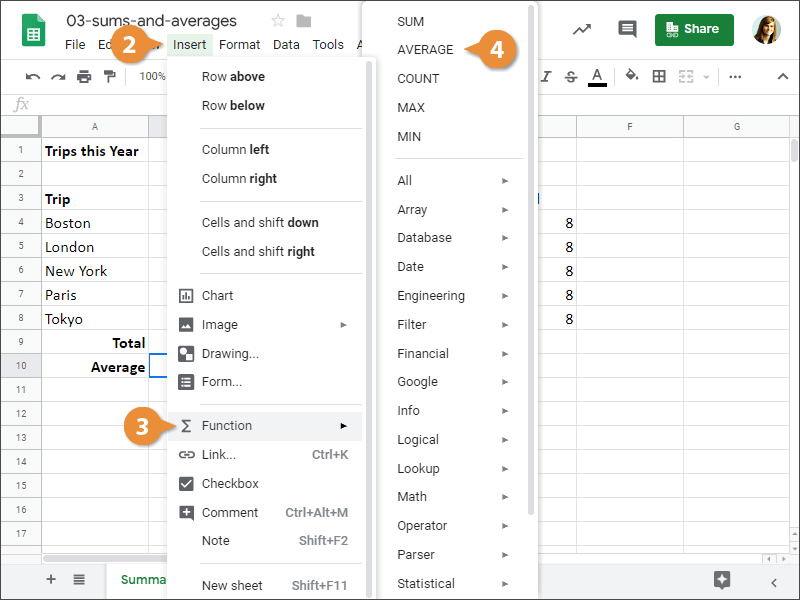5. Select the cells you want to find the average of.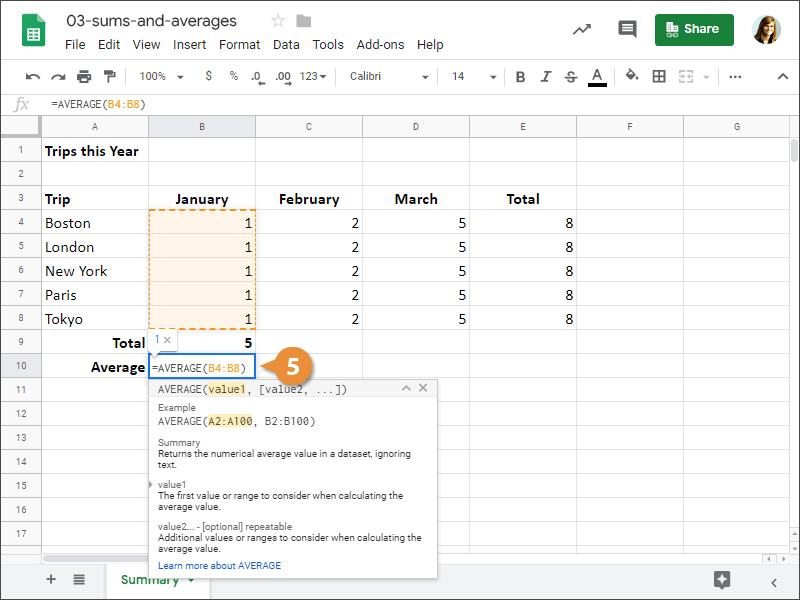6. Press Enter.

The average of the cell range is displayed in the cell.